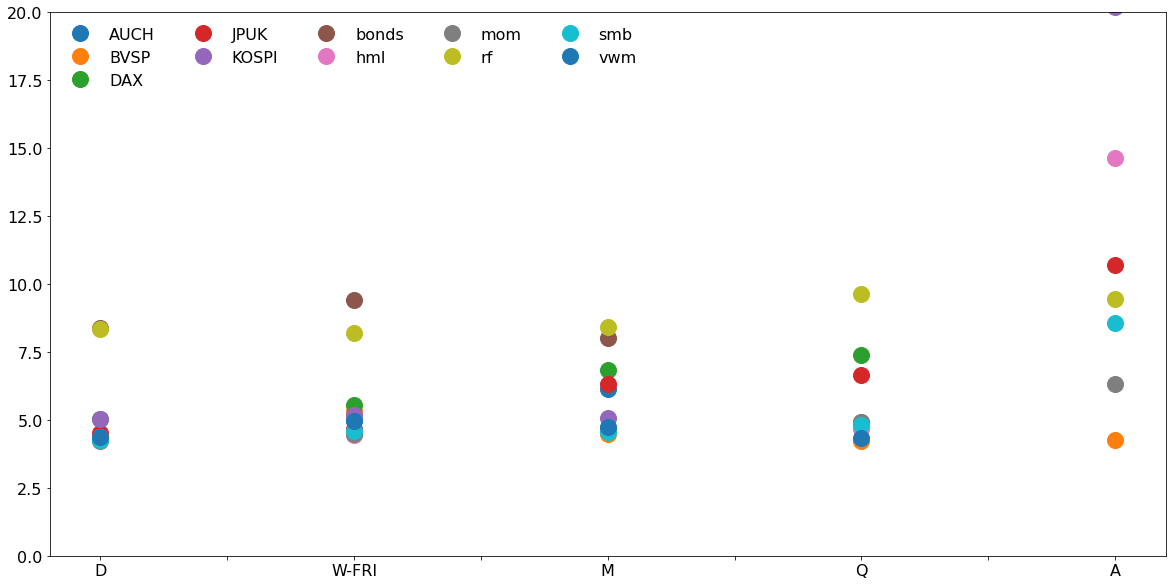# Computational Assignment 1¶

This file provides a walk-through of some of the analysis methods that could have been used in the assignment.

I also highlight some code patterns that might help you in future assignments.

## Data¶

### FRED Data¶

• Import the data
• Convert missing values to NaN using to_numeric
• Construct cross-rates
• Note: The Japanese rate is Dollar/Yen by convention. The other are Foreign/Dollar.

#### Remarks¶

1. Reading data with parse_dates=True and the index_col set parses the dates and sets them as the index. This is usually a good idea.
2. pd.to_numeric can be used to convert a Series with strange missing value markerts to NaN.
In :
import pandas as pd

uk = pd.read_csv("DEXUSUK.csv", index_col="DATE", parse_dates=True).iloc[:, 0]
jp = pd.read_csv("DEXJPUS.csv", index_col="DATE", parse_dates=True).iloc[:, 0]
ch = pd.read_csv("DEXSZUS.csv", index_col="DATE", parse_dates=True).iloc[:, 0]
au = pd.read_csv("DEXUSAL.csv", index_col="DATE", parse_dates=True).iloc[:, 0]

uk = pd.to_numeric(uk, errors="coerce")
ch = pd.to_numeric(ch, errors="coerce")
au = pd.to_numeric(au, errors="coerce")
jp = pd.to_numeric(jp, errors="coerce")

jpuk = jp * uk
auch = au / ch
jpuk.name = "JPUK"
auch.name = "AUCH"


### French Data¶

The CSV files were cleaned prior to import.

#### Remarks¶

1. pd.to_datetime is a simple way to convert string or integer dates to pandas dates. The format argument accepts many different styles. The most important are

• %Y: 4-digit year
• %m: 2-digit month
• %d : 2-digit day
In :
factors = pd.read_csv("ff-factors.csv")
factors["Date"] = pd.to_datetime(factors.Date, format="%Y%m%d")
mom["Date"] = pd.to_datetime(mom.Date, format="%Y%m%d")
factors = factors.set_index("Date")
mom = mom.set_index("Date")
ff_returns = pd.concat([factors, mom], axis=1)

# Add RF back to VWM, optional
# ff_returns["Mkt-RF"] = ff_returns["Mkt-RF"] + ff_returns["RF"].values

# Price index construction
ff = (1 + ff_returns / 100).cumprod()
ff.columns = ["vwm", "smb", "hml", "rf", "mom"]
ff = ff[["vwm", "smb", "hml", "rf", "mom"]]


### Yahoo Data¶

In :
kospi = pd.read_csv("^KS11.csv", index_col="Date", parse_dates=True)["Adj Close"]
kospi.name = "KOSPI"
bvsp.name = "BVSP"
dax.name = "DAX"
bond_fund.name = "bonds"


### Merge Data¶

Here I combine all series into a DataFrame which will let me loop over columns easily.

#### Remarks¶

1. String dates of the form "YYYY", "YYYY-mm", "YYYY-mm-dd" can be used to slice pandas Series and DataFrames that have DatetimeIndex indices. The slice includes all values in the range, including the end points. For example, [:"2019"] includes all data up-to-and-including December 31, 2019.
2. Merging data can simplify repeated tasks since it is possible to loop over the columns in a DataFrame using the syntax:
for col in df:
one_column = f[col]
# do something useful with the columns

In :
combined = pd.concat([ff, kospi, bvsp, dax, jpuk, auch, bond_fund], axis=1)
combined = combined[:"2019"]
combined.tail()

Out:
vwm smb hml rf mom KOSPI BVSP DAX JPUK AUCH bonds
2019-12-25 NaN NaN NaN NaN NaN NaN NaN NaN NaN NaN NaN
2019-12-26 359.871254 1.923631 29.302372 21.155412 339.782285 2197.929932 117203.0 NaN 142.647769 0.707484 10.824861
2019-12-27 359.511383 1.913628 29.281861 21.156893 341.039479 2204.209961 116534.0 13337.110352 143.296230 0.716354 10.834646
2019-12-30 357.462168 1.916882 29.457552 21.158374 341.175895 2197.669922 115964.0 13249.009766 143.028900 0.723778 10.834646
2019-12-31 358.463062 1.916690 29.495846 21.159855 339.538251 NaN NaN NaN 144.194223 0.726465 10.839552

# summary_statistics¶

One of the required function for the autograder is presented below. I build a DataFrame from Series.

#### Remarks¶

1. Dictionaries (i.e., d = {}) are important for writing efficient code. They are also easy to convert to pandas Series and dictionaries of Series can be easily converted in DataFrames. This is exactly how I build the DataFrame below. Dictionaries can also be looped over using the same syntax as a DataFrame:
d = {"a": 1, "b": 2, "c": 3}
for key in d:
value = d[key]
# do something useful

In :
import numpy as np

def summary_statistics(prices):
prices = prices.dropna()
values = {}
# A better approach to scale would be to use a dictionary
scales = {"D": 252, "W-FRI": 52, "M": 12, "Q": 4, "A": 1}
for freq in ("D", "W-FRI", "M", "Q", "A"):
resample = prices.resample(freq).last()
returns = resample.dropna().pct_change().dropna()
# The better approack
# scale = scaled[freq]
if freq == "D":
scale = 252
elif freq == "W-FRI":
scale = 52
elif freq == "M":
scale = 12
elif freq == "Q":
scale = 4
else:
scale = 1
# A dictionary to convert to a Series
moments = {
"mean": scale * returns.mean(),
"std": np.sqrt(scale) * returns.std(),
"skew": returns.skew(),
"kurt": returns.kurt() + 3,
}
# Store the Series in another dictionary.
values[freq] = pd.Series(moments)
all_freq = pd.DataFrame(values)
all_freq.columns = ["Daily", "Weekly", "Monthly", "Quarterly", "Annual"]
return all_freq

summary_statistics(combined.vwm)

Out:
Daily Weekly Monthly Quarterly Annual
mean 0.074359 0.077469 0.079803 0.085298 0.083965
std 0.168455 0.171430 0.184345 0.220219 0.198535
skew -0.120938 -0.358674 0.193515 1.832394 -0.303247
kurt 19.633944 9.156717 10.997113 20.132500 3.048339

Here I collect all of the analyses into groups by frequency by storing them a dictionary

In :
def group_by_freq(prices, series):
# Empty dictionary where each will hold another dictionary
grouped = {}
for col in series:
temp = summary_statistics(prices[col])
for freq in temp:
single = temp[freq]
# Check if the key exists, if not, create it with an empty dictionary
if freq not in grouped:
grouped[freq] = {}
# Store the Series as an element in the grouped[freq] dictionary
grouped[freq][col] = single
for col in grouped:
# Convert each inner dictionary to a DataFrame
grouped[col] = pd.DataFrame(grouped[col])
return grouped

by_freq = group_by_freq(
combined,
["vwm", "smb", "hml", "mom", "DAX", "BVSP", "KOSPI", "AUCH", "JPUK", "bonds"],
)

by_freq["Daily"]

Out:
vwm smb hml mom DAX BVSP KOSPI AUCH JPUK bonds
mean 0.074359 0.010963 0.038946 0.066841 0.104627 0.385818 0.085484 0.036962 -0.029825 0.037948
std 0.168455 0.092420 0.092934 0.118254 0.219834 0.355286 0.271989 0.177073 0.115650 0.039202
skew -0.120938 -0.754706 0.731388 -1.594806 -0.081570 0.950318 -0.025284 -0.041135 -0.780582 0.075773
kurt 19.633944 25.690942 18.768387 29.870722 8.797502 17.768665 8.892787 21.146994 14.505053 4.367833
In :
by_freq["Monthly"]

Out:
vwm smb hml mom DAX BVSP KOSPI AUCH JPUK bonds
mean 0.079803 0.012764 0.043009 0.074526 0.101791 0.404131 0.086443 0.037605 -0.029404 0.038846
std 0.184345 0.106450 0.114842 0.151961 0.202885 0.437562 0.276574 0.182923 0.118606 0.034419
skew 0.193515 1.122333 1.292989 -0.916640 -0.520413 2.298289 0.851238 0.116887 -0.610010 -0.206248
kurt 10.997113 13.697603 12.403212 11.418075 5.104647 15.307200 8.567472 5.804064 5.584841 4.494305
In :
by_freq["Annual"]

Out:
vwm smb hml mom DAX BVSP KOSPI AUCH JPUK bonds
mean 0.083965 0.014387 0.044961 0.077053 0.111510 0.607236 0.128481 0.033919 -0.025877 0.040159
std 0.198535 0.117578 0.130961 0.154629 0.233566 2.082155 0.311787 0.185100 0.135514 0.028089
skew -0.303247 0.229972 0.359051 -0.733467 -0.685109 4.759770 0.112506 0.568858 0.288654 -0.432868
kurt 3.048339 4.313512 3.563743 5.582957 2.961143 26.549166 3.370362 2.423933 3.896373 3.017251

There is another way to do the same, which is to use a MultiIndex where we have to levels to the index, freq and col.

In :
all_stats = []
for col in ["vwm", "smb", "hml", "mom", "DAX", "BVSP", "KOSPI", "AUCH", "JPUK", "bonds"]:
stats = summary_statistics(combined[col])
stats.columns = pd.MultiIndex.from_tuples([(freq,col) for freq in stats])
all_stats.append(stats)
all_stats = pd.concat(all_stats,1)
all_stats.loc[:,"Daily"]

Out:
vwm smb hml mom DAX BVSP KOSPI AUCH JPUK bonds
mean 0.074359 0.010963 0.038946 0.066841 0.104627 0.385818 0.085484 0.036962 -0.029825 0.037948
std 0.168455 0.092420 0.092934 0.118254 0.219834 0.355286 0.271989 0.177073 0.115650 0.039202
skew -0.120938 -0.754706 0.731388 -1.594806 -0.081570 0.950318 -0.025284 -0.041135 -0.780582 0.075773
kurt 19.633944 25.690942 18.768387 29.870722 8.797502 17.768665 8.892787 21.146994 14.505053 4.367833
In :
all_stats.loc[:,"Weekly"]

Out:
vwm smb hml mom DAX BVSP KOSPI AUCH JPUK bonds
mean 0.077469 0.011146 0.041316 0.071878 0.102941 0.378262 0.082640 0.036648 -0.028877 0.038887
std 0.171430 0.091119 0.102372 0.137378 0.211765 0.351635 0.270248 0.176249 0.121044 0.037188
skew -0.358674 0.084792 1.102395 -1.389963 -0.350936 0.632277 -0.144330 -0.093208 -0.925644 -0.396488
kurt 9.156717 13.159411 16.190505 15.522252 6.880258 7.520713 8.057720 9.060139 11.877077 4.108810

I look at monthly stats for each decade. One could look at other sampling frequencies or decade definitions.

#### Remarks¶

1. I use a property of pandas Series and DataFrames that have DatetimeIndex that let blocks be selected using string dates, e.g., data["1950":"1959"] which selects all data with years in 1950 to 1959, inclusive.
In :
def monthly_stats(prices, decade_starts):
values = {}
return pd.DataFrame(values)

monthly_stats(combined.vwm, np.arange(1930, 2020, 10))

Out:
1930 1940 1950 1960 1970 1980 1990 2000 2010
mean 0.050890 0.102658 0.156654 0.056932 0.019354 0.079837 0.136544 -0.012911 0.135590
std 0.363584 0.154697 0.112423 0.124866 0.167957 0.167989 0.135518 0.166490 0.129452
skew 0.731872 -1.331744 -0.279851 -0.264714 0.051208 -0.911132 -0.792294 -0.636636 -0.395563
kurt 5.819518 7.632213 2.574932 3.379399 4.018050 7.345798 5.471178 3.689987 3.645049

There are many ways to compare the statistics. I have plotted the statistics in all year 10-year blocks (i.e., 1930-1939, 1931-1940, ...) and the long-run value of the statistic.

In :
import matplotlib.pyplot as plt

plt.rc("figure", figsize=(20, 10))
plt.rc("font", size=16)

full = {}
for col in series:
data = combined[col].dropna()
first = (data.index.year // 10 + 1) * 10
last = (data.index[-1].year // 10 + 1) * 10
first = data.index.year
else:
full[col] = summary_statistics(data)["Monthly"]
fig, ax = plt.subplots(4, 1)
x = i * np.ones_like(this_stat)
ax[j].plot(x, this_stat, linewidth=0, marker="o", markersize=12, alpha=0.5)
ax[j].plot(i, full[col].loc[stat], linewidth=0, marker="s", markersize=24)
ax[j].set_title(stat)
if j < (len(decade_stats) - 1):
ax[j].set_xticklabels([])
else: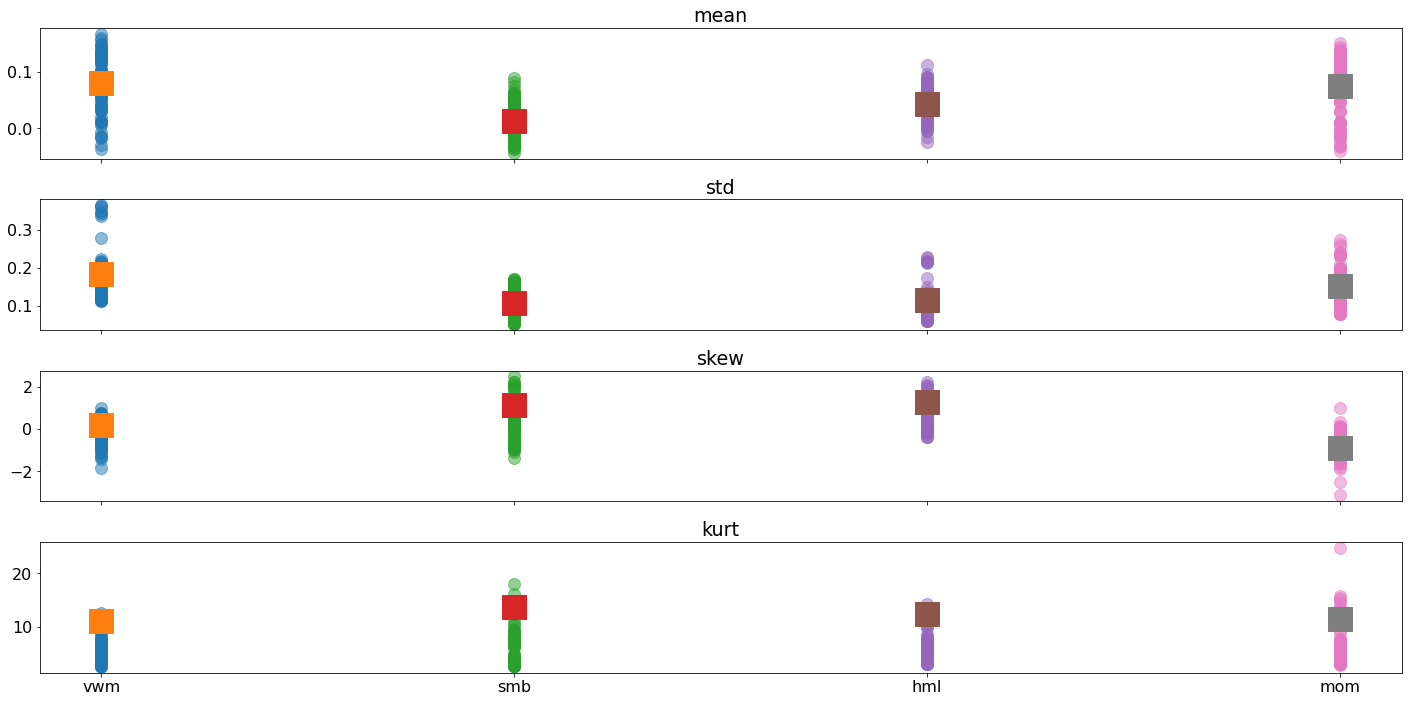In :
plot_monthly(["DAX", "KOSPI", "BVSP", "bonds"], all_decades=True)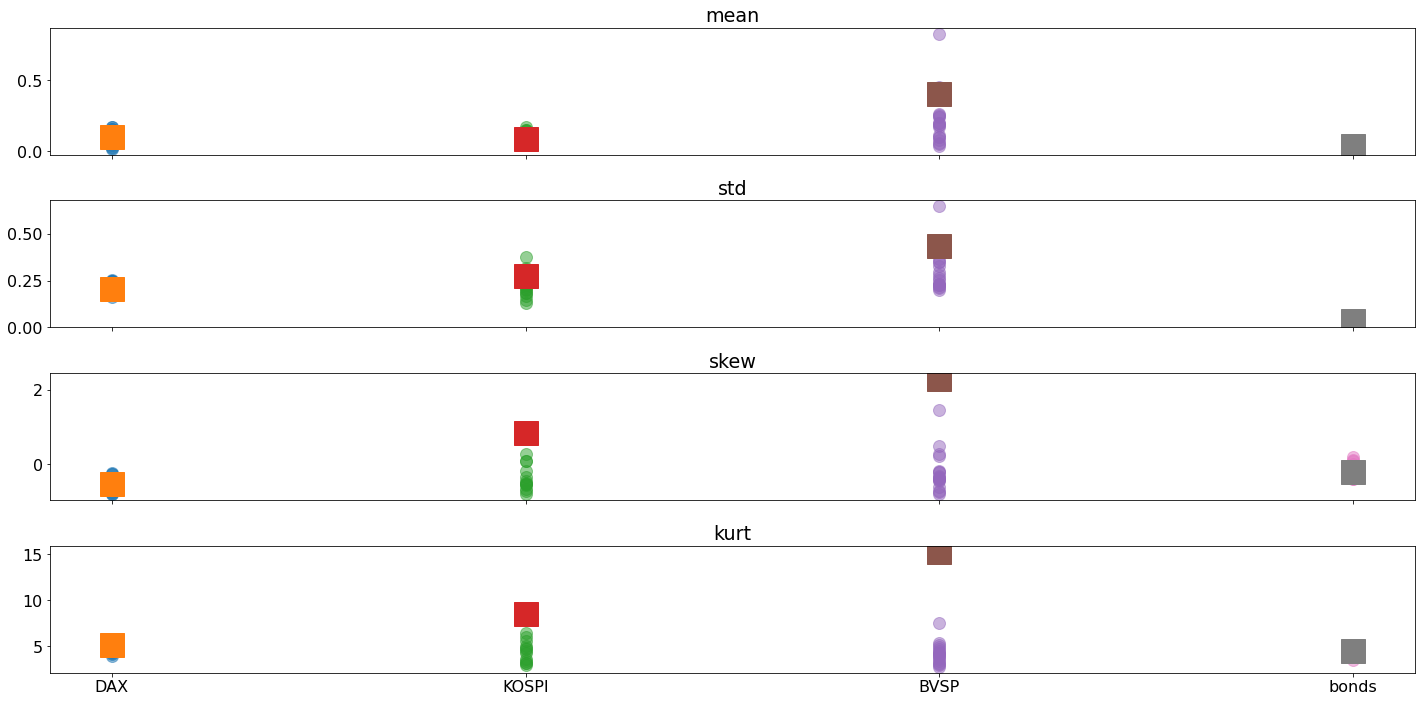In :
plot_monthly(["JPUK", "AUCH"], all_decades=True)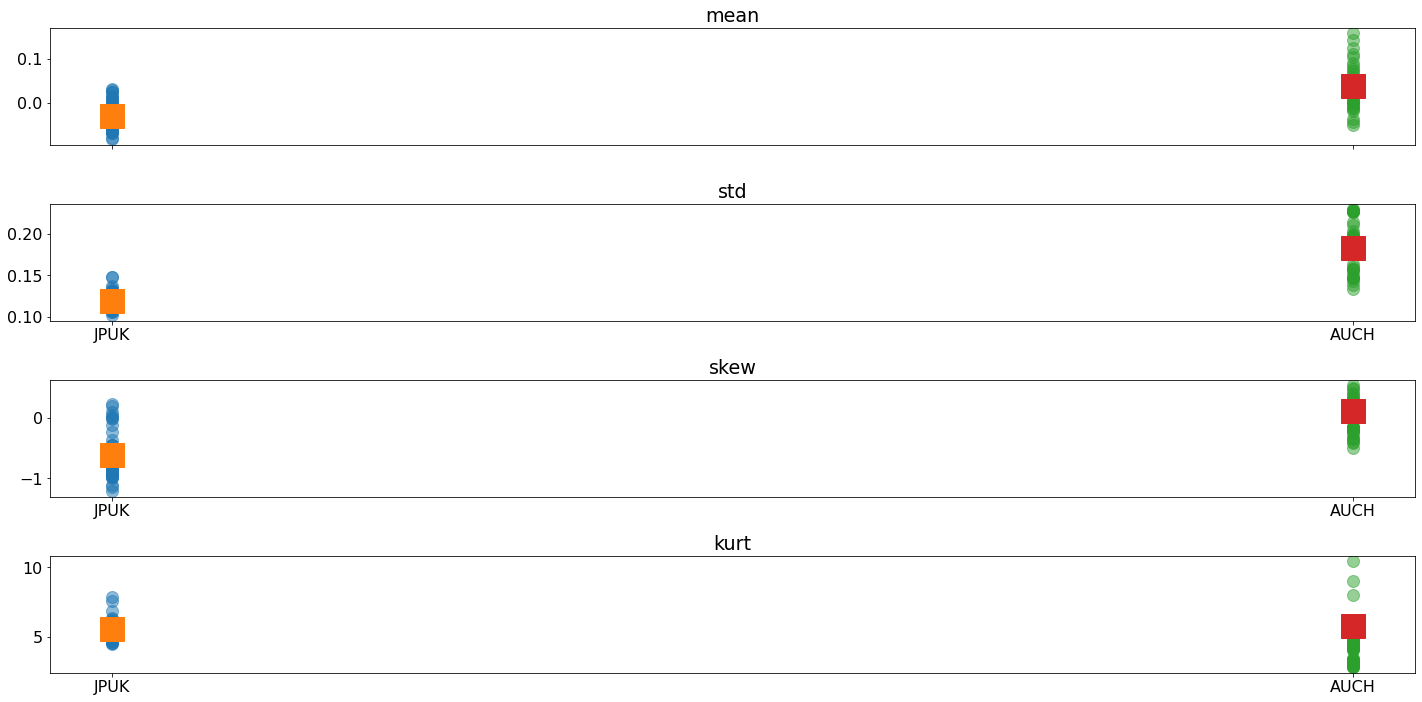Finally I compute the DoF for all frequencies using both the MLE and the correct moment-based estimator,

$$\hat{\nu}_{\text{Mom}} = \frac{4\kappa-6}{\kappa-3}, \kappa > 3$$

The MLE uses the same approach as in the companion course.

In :
from scipy.special import gammaln
from scipy.optimize import minimize

def std_t_loglik(nu, x):
# These are fixed for now
mu = 0
sigma2 = 1
sigma = np.sqrt(sigma2)

a = gammaln((nu + 1) / 2)
b = gammaln(nu / 2)
c = np.sqrt(np.pi * (nu - 2))
d = (nu + 1) / 2
e = (x - mu) ** 2
f = sigma2 * (nu - 2)

loglik = a - b - np.log(c) - np.log(sigma) - d * np.log(1 + e / f)
return -(loglik.sum())

def moment_nu(rets):
kappa = rets.kurtosis() + 3
if kappa <= 3:
return float(np.inf)
return float((4 * kappa - 6) / (kappa - 3))


The DoF values are computed from the functions and then stored in a dictionary. The key to the dictionary is a 2-element tuple of the form (column, frequency) which pandas automatically converts to a MultiIndex. This simplifies conversion later.

In :
dof = {}
for col in combined:
for freq in ("D", "W-FRI", "M", "Q", "A"):
key = (col, freq)
rets = (
combined[col].dropna().resample(freq).last().dropna().pct_change().dropna()
)
std_rets = (rets - rets.mean()) / rets.std()
starting_val = np.array()
opt = minimize(
std_t_loglik,
starting_val,
args=(std_rets,),
bounds=[(2.05, 100)],
options={"disp": False},
)
dof[key] = [float(np.squeeze(opt.x)), moment_nu(rets)]
dof = pd.DataFrame(dof, index=["MLE", "Moment"]).T


Out:
MLE Moment
vwm D 2.903435 4.360708
W-FRI 3.635643 4.974545
M 3.840701 4.750271
Q 3.200565 4.350212
A 100.000000 128.124517
smb D 2.977160 4.264423
W-FRI 3.616538 4.590585
M 4.217550 4.560873
Q 5.539519 4.814348
A 5.718195 8.567907
hml D 2.902944 4.380508
W-FRI 3.071014 4.454873
M 3.336994 4.638080
Q 3.444944 4.656433
A 21.478546 14.643151

### Unstacking the values¶

We can select the MLE values and then use unstack to transform a 2-level MultiIndex in the a rectangualr table. This will use the "outer" index value (column) as the new index, and the "inner" column values as the column

In :
mle = dof.MLE.unstack()[["D","W-FRI","M","Q","A"]]
mle

Out:
D W-FRI M Q A
AUCH 3.719821 4.557508 5.020495 15.669813 100.000000
BVSP 3.451807 3.599981 2.847346 2.271698 2.152317
DAX 3.747828 5.061805 5.147151 4.146419 100.000000
JPUK 3.560871 3.955905 4.818283 7.060872 9.413998
KOSPI 2.937987 3.267547 3.453366 2.790256 52.871615
bonds 7.227888 7.952128 6.508588 100.000000 100.000000
hml 2.902944 3.071014 3.336994 3.444944 21.478546
mom 2.760501 2.979109 3.077491 3.297278 4.223921
rf 11.266749 11.072139 11.545661 13.062032 13.279122
smb 2.977160 3.616538 4.217550 5.539519 5.718195
vwm 2.903435 3.635643 3.840701 3.200565 100.000000

### Plotting¶

We can plot them by frequency to see if there is any obvious pattern.

In :
ax = mle.T.plot(legend=False,marker="o",markersize=16,linewidth=0)
ax.set_ylim(0,20)
_ = ax.legend(frameon=False,ncol=5)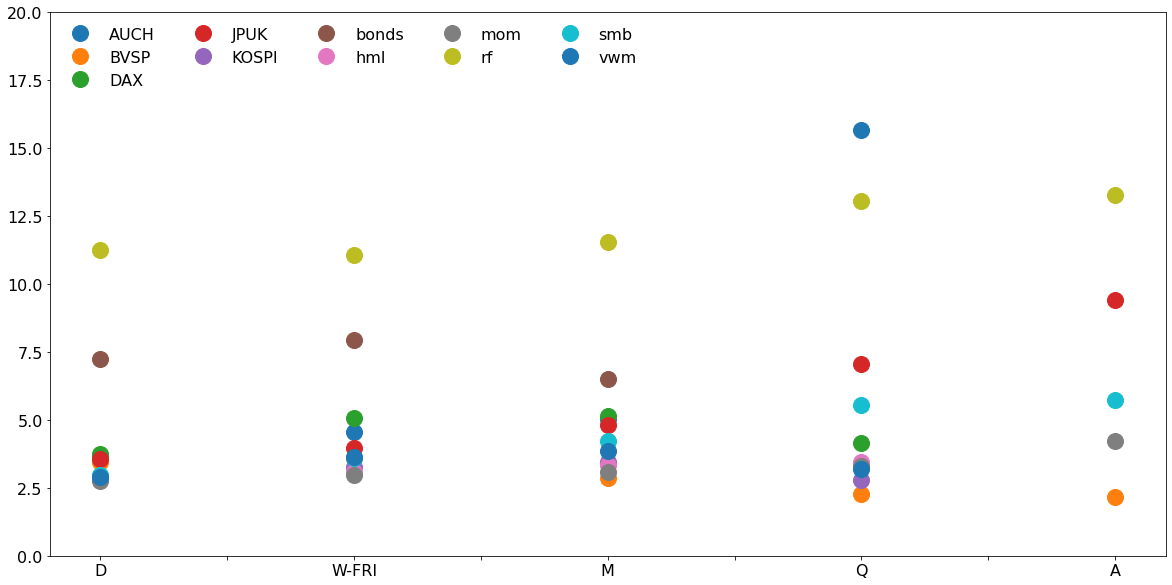In :
moment = dof.Moment.unstack()[["D","W-FRI","M","Q","A"]]
moment

Out:
D W-FRI M Q A
AUCH 4.330633 4.990076 6.139751 22.852496 inf
BVSP 4.406266 5.327224 4.487519 4.230676 4.254786
DAX 5.034928 5.546289 6.850834 7.385049 inf
JPUK 4.521510 4.675898 6.321226 6.644074 10.693645
KOSPI 5.018194 5.186305 5.077688 4.929423 20.200356
bonds 8.386499 9.411206 8.015244 inf 351.813073
hml 4.380508 4.454873 4.638080 4.656433 14.643151
mom 4.223291 4.479147 4.712752 4.937449 6.322919
rf 8.329772 8.207837 8.402670 9.617265 9.434982
smb 4.264423 4.590585 4.560873 4.814348 8.567907
vwm 4.360708 4.974545 4.750271 4.350212 128.124517
In :
ax = moment.T.plot(legend=False,marker="o",markersize=16,linewidth=0)
ax.set_ylim(0,20)
_ = ax.legend(frameon=False,ncol=5,loc=2)